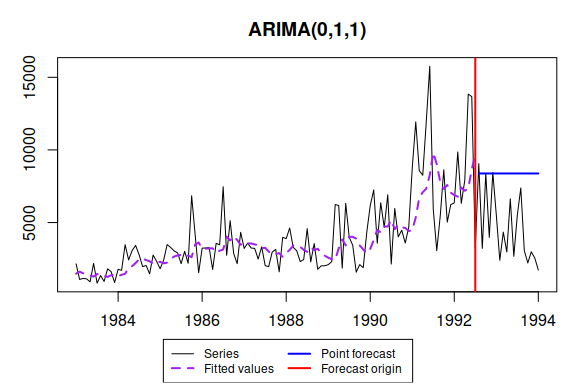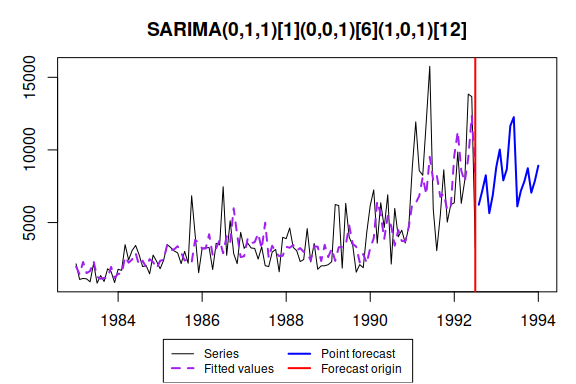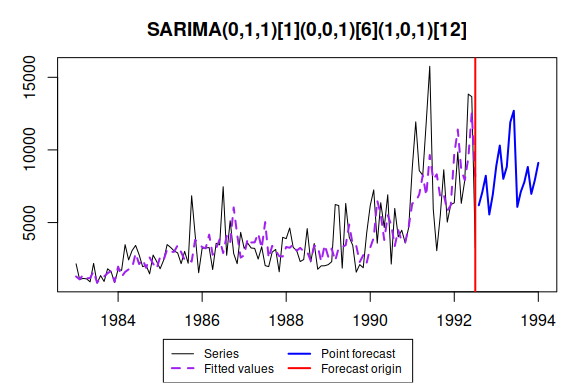# ssarima() - State-Space ARIMA

#### 2020-06-16

SSARIMA stands for “State-space ARIMA” or “Several Seasonalities ARIMA”. Both names show what happens in the heart of the function: it constructs ARIMA in a state-space form and allows to model several (actually more than several) seasonalities. ssarima() is a function included in smooth package. This vignette covers ssarima() and auto.ssarima() functions. For more details about the underlying model, read (Svetunkov and Boylan 2019).

As usual, we will use data from Mcomp package, so it is advised to install it.

require(smooth)
require(Mcomp)

The default call constructs ARIMA(0,1,1):

ssarima(M3$N2457, h=18, silent=FALSE) ## Time elapsed: 0.04 seconds ## Model estimated: ARIMA(0,1,1) ## Matrix of MA terms: ## Lag 1 ## MA(1) -0.7941 ## Initial values were produced using backcasting. ## ## Loss function type: MSE; Loss function value: 4401089.934 ## Error standard deviation: 2116.361 ## Sample size: 115 ## Number of estimated parameters: 2 ## Number of degrees of freedom: 113 ## Information criteria: ## AIC AICc BIC BICc ## 2089.553 2089.660 2095.042 2095.297 ## ## Forecast errors: ## MPE: -132.4%; sCE: -1695.9%; Bias: -95.3%; MAPE: 133.3% ## MASE: 2.348; sMAE: 96.3%; sMSE: 123.3%; rMAE: 1.954; rRMSE: 1.639Some more complicated model can be defined using parameter orders the following way: ssarima(M3$N2457, orders=list(ar=c(0,1),i=c(1,0),ma=c(1,1)), lags=c(1,12), h=18)
## Time elapsed: 0.17 seconds
## Model estimated: SARIMA(0,1,1)(1,0,1)
## Matrix of AR terms:
##       Lag 12
## AR(1) 0.8298
## Matrix of MA terms:
##         Lag 1  Lag 12
## MA(1) -0.8141 -0.3689
## Initial values were produced using backcasting.
##
## Loss function type: MSE; Loss function value: 3618199.0582
## Error standard deviation: 1936.126
## Sample size: 115
## Number of estimated parameters: 4
## Number of degrees of freedom: 111
## Information criteria:
##      AIC     AICc      BIC     BICc
## 2071.027 2071.390 2082.007 2082.869
##
## Forecast errors:
## MPE: -133.1%; sCE: -1582.1%; Bias: -82.2%; MAPE: 139.9%
## MASE: 2.502; sMAE: 102.6%; sMSE: 149%; rMAE: 2.082; rRMSE: 1.802

This would construct us seasonal ARIMA(0,1,1)(1,0,1)$$_{12}$$.

We could try selecting orders manually, but this can also be done automatically via auto.ssarima() function:

auto.ssarima(M3$N2457$x, h=18)
## Time elapsed: 4.58 seconds
## Model estimated: SARIMA(0,1,2)(0,0,3)
## Matrix of MA terms:
##         Lag 1 Lag 12
## MA(1) -0.5942 0.3920
## MA(2) -0.2769 0.6312
## MA(3)  0.0000 0.0494
## Initial values were produced using backcasting.
##
## Loss function type: MSE; Loss function value: 3183490.065
## Error standard deviation: 1832.683
## Sample size: 115
## Number of estimated parameters: 6
## Number of degrees of freedom: 109
## Information criteria:
##      AIC     AICc      BIC     BICc
## 2060.307 2061.085 2076.777 2078.622

Automatic order selection in SSARIMA with optimised initials does not work well and in general is not recommended. This is partially because of the possible high number of parameters in some models and partially because of potential overfitting of first observations when non-zero order of AR is selected. This problem can be seen on example of another time series (which has complicated seasonality):

auto.ssarima(M3$N1683$x, h=18, initial="backcasting")
## Time elapsed: 6.98 seconds
## Model estimated: SARIMA(0,0,3)(3,1,3)
## Matrix of AR terms:
##        Lag 12
## AR(1) -0.3158
## AR(2) -0.4372
## AR(3)  0.0313
## Matrix of MA terms:
##        Lag 1  Lag 12
## MA(1) 0.0994 -0.6873
## MA(2) 0.1297  0.7312
## MA(3) 0.2224 -0.9547
## Initial values were produced using backcasting.
##
## Loss function type: MSE; Loss function value: 76412.9453
## Error standard deviation: 290.1899
## Sample size: 108
## Number of estimated parameters: 10
## Number of degrees of freedom: 98
## Information criteria:
##      AIC     AICc      BIC     BICc
## 1540.833 1543.101 1567.654 1572.964
auto.ssarima(M3$N1683$x, h=18, initial="optimal")
## Time elapsed: 9.4 seconds
## Model estimated: ARIMA(0,0,3) with constant
## Matrix of MA terms:
##        Lag 1
## MA(1) 0.3558
## MA(2) 0.2926
## MA(3) 0.3104
## Constant value is: 3786.8136
## Initial values were optimised.
##
## Loss function type: MSE; Loss function value: 169345.8886
## Error standard deviation: 427.6606
## Sample size: 108
## Number of estimated parameters: 8
## Number of degrees of freedom: 100
## Information criteria:
##      AIC     AICc      BIC     BICc
## 1622.778 1624.233 1644.235 1647.640

As can be seen from the second graph, ssarima with optimal initial does not select seasonal model and reverts to ARIMA(0,0,3) with constant. In theory this can be due to implemented order selection algorithm, however if we estimate all the model in the pool separately, we will see that this model is optimal for this time series when this type of initials is used.

A power of ssarima() function is that it can estimate SARIMA models with multiple seasonalities. For example, SARIMA(0,1,1)(0,0,1)_6(1,0,1)_12 model can be estimated the following way:

ssarima(M3$N2457$x, orders=list(ar=c(0,0,1),i=c(1,0,0),ma=c(1,1,1)), lags=c(1,6,12), h=18, silent=FALSE)
## Time elapsed: 0.46 seconds
## Model estimated: SARIMA(0,1,1)(0,0,1)(1,0,1)
## Matrix of AR terms:
##       Lag 12
## AR(1) 0.7505
## Matrix of MA terms:
##         Lag 1   Lag 6  Lag 12
## MA(1) -0.8038 -0.2056 -0.3107
## Initial values were produced using backcasting.
##
## Loss function type: MSE; Loss function value: 3448766.7318
## Error standard deviation: 1898.823
## Sample size: 115
## Number of estimated parameters: 5
## Number of degrees of freedom: 110
## Information criteria:
##      AIC     AICc      BIC     BICc
## 2067.512 2068.062 2081.236 2082.542It probably does not make much sense for this type of data, it would make more sense on high frequency data (for example, taylor series from forecast package). However, keep in mind that multiple seasonal ARIMAs are very slow in estimation and are very capricious. So it is really hard to obtain an appropriate and efficient multiple seasonal ARIMA model.

Now let’s introduce some artificial exogenous variables:

x <- cbind(rnorm(length(M3$N2457$x),50,3),rnorm(length(M3$N2457$x),100,7))

If we save model:

ourModel <- auto.ssarima(M3$N2457$x, h=18, holdout=TRUE, xreg=x, updateX=TRUE)

we can then reuse it:

ssarima(M3$N2457$x, model=ourModel, h=18, holdout=FALSE, xreg=x, updateX=TRUE, interval=TRUE)
## Time elapsed: 1.35 seconds
## Model estimated: SARIMAX(0,0,3)(0,1,3) with drift
## Matrix of MA terms:
##         Lag 1  Lag 12
## MA(1)  0.3849 -0.9459
## MA(2)  0.1986  0.4509
## MA(3) -0.1363  0.1829
## Constant value is: 104.5742
## Initial values were provided by user.
## Xreg coefficients were estimated in a crazy style
##
## Loss function type: MSE; Loss function value: 4671171.2688
## Error standard deviation: 2170.748
## Sample size: 115
## Number of estimated parameters: 1
## Number of provided parameters: 54
## Number of degrees of freedom: 114
## Information criteria:
##      AIC     AICc      BIC     BICc
## 2094.402 2094.437 2097.147 2097.231
##
## 95% parametric prediction interval was constructed

Finally, we can combine several SARIMA models:

ssarima(M3$N2457$x, h=18, holdout=FALSE, interval=TRUE, combine=TRUE)
## Time elapsed: 0.03 seconds
## Model estimated: ARIMA(0,1,1)
## Matrix of MA terms:
##         Lag 1
## MA(1) -0.7941
## Initial values were produced using backcasting.
##
## Loss function type: MSE; Loss function value: 4401089.934
## Error standard deviation: 2116.361
## Sample size: 115
## Number of estimated parameters: 2
## Number of degrees of freedom: 113
## Information criteria:
##      AIC     AICc      BIC     BICc
## 2089.553 2089.660 2095.042 2095.297
##
## 95% parametric prediction interval was constructed

# MSARIMA

While SSARIMA is flexible, it is not very fast. In fact, it cannot handle high frequency data well and most probably will take ages to estimate the parameter and produce forecasts. The MSARIMA model (Multiple Seasonal ARIMA) is formulated in a different state-space form, which reduces the size of transition matrix, significanltly reducing the computational time for cases with high frequency data. However, due to that formulation, the only way to initialise the model is using backcasting.

There are auto.msarima() and msarima() function in the package, that do things similar to auto.ssarima() and ssarima(). Here’s just one example of what can be done with it:

msarima(M3$N2457$x, orders=list(ar=c(0,0,1),i=c(1,0,0),ma=c(1,1,1)),lags=c(1,6,12),h=18, silent=FALSE)
## Time elapsed: 0.23 seconds
## Model estimated: SARIMA(0,1,1)(0,0,1)(1,0,1)
## Matrix of AR terms:
##       Lag 12
## AR(1) 0.8154
## Matrix of MA terms:
##         Lag 1   Lag 6  Lag 12
## MA(1) -0.8013 -0.1978 -0.3654
## Initial values were produced using backcasting.
##
## Loss function type: MSE; Loss function value: 3475705.7785
## Error standard deviation: 1906.225
## Sample size: 115
## Number of estimated parameters: 5
## Number of degrees of freedom: 110
## Information criteria:
##      AIC     AICc      BIC     BICc
## 2068.406 2068.957 2082.131 2083.437The forecasts of the two models will differ due to the different state space form.